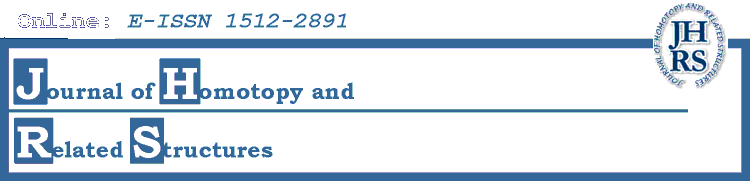# Editors' Interests

• Claude Cibils
Hochschild (co)homology, representations of quantum groups, and associative algebras.
• Frederick Cohen
Algebraic topology, group theory, surface theory, cohomology of groups, applications to physics.
• Guillermo Cortinas
K-theory, cyclic homology, noncommutative geometry.
• Diarmuid Crowley
Differential topology and Algebraic topology; the surgery classification of manifolds. Especially 7- manifolds and G2-structures, almost contact structures, embddings in co-dimension > 3, mapping class groups in high dimensions and exotic spheres and the Gromoll filtration.
• Christopher Douglas
Algebraic and geometric topology
• Bjorn Ian Dundas
Stable equivariant homotopy theory, algebraic K-theory, homotopy type theory
• Peter Eccles
Homotopy theory, cobordism theory and relationship between them.
• Graham Ellis
Homological and homotopical algebra.
• Benson Farb
Geometric topology, group actions, discrete subgroups of Lie groups.
• Marino Gran
Categorical algebra, Galois theory, universal algebra.
Homological and K-theoretic aspects of toric varieties and polytopes
• Jens Hornbostel
Motivic homotopy theory, algebraic K-theory, stable homotopy theory.
• Johannes Huebschmann
Homological algebra, algebraic topology, topological methods in physics.
• Hvedri Inassaridze
K-theory, homological and homotopical algebra, noncommutative geometry.
• Nick Inassaridze
Homological and homotopical algebra, cyclic homology.
• Stefan Jackowski
Homotopy theory, classifying spaces, group actions, homological algebra, relationships and applications across various fields of algebra and topology.
• George Janelidze
Category theory, homological algebra, Galois theory.
• Tyler Lawson
Stable homotopy theory.
• Haynes Miller
Homotopy theory, relation between elliptic curves and homotopy theory, the Steenrod algebra, the homotopy theory of Lie groups.
• Ralf Meyer
K-theory and bivariant K-theory, non-commutative geometry, cyclic homology, homological algebra.
• Krzysztof Pawałowski
Transformation groups, more specifically, group actions on manifolds.
• Tim Porter
Algebraic homotopy, homotopy coherence, strong shape theory and proper homotopy theory, global actions, groupoid atlases, abstract homotopy theory.
• Geoffrey Powell
Unstable and stable homotopy theory; (un)stable modules over the Steenrod algebra; (co)homology operations; functor categories; homotopical and derived geometry.
• Stewart Priddy
Stable homotopy theory.
• Martin Raussen
Applications of homotopy theory in computer science.
• Ulf Rehmann
Linear algebraic groups and related structures.
• Emily Riehl
Category theory, homotopy theory, Homotopy type theory.
• Jonathan Rosenberg
Topology and geometry of manifolds, index theory, noncommutative geometry.
• Jiri Rosicky
Category theory, homotopy theories, homotopy categories.
• Thomas Schick
Geometric topology, K-theory in particular of operator algebras.
• Ross Staffeldt
Algebraic topology, algebraic K-theory.
• James Stasheff
Higher homotopy algebra, operads, cohomological physics, homotopical physics.
• Ross Street
Enriched category theory, higher-dimensional category theory.
Homotopy properties of configuration spaces, Adams-Novikov spectral sequence, cobordism.
• Gabriele Vezzosi
Algebraic Geometry, Derived Algebraic Geometry, Higher Categories.
• Christian Voigt
Operator K-theory, Hochschild and cyclic homology, Hopf algebras, quantum groups
• Charles Weibel
Algebraic K-theory, motivic cohomology, cyclic homology, algebraic geometry, homological algebra.
• Craig Westerland
Stable and chromatic homotopy theory, homotopy theory of configuration and moduli spaces, and homotopy theoretic techniques in arithmetic geometry.
• Simon Willerton
Magnitude of metric spaces, topological quantum field theory, category theory.
• Steven Weintraub
Differential topology, algebraic geometry.
• Scott O. Wilson
Geometry and topology of manifolds, homotopy algebra, differential cohomology.
• W.Stephen Wilson
Algebraic topology, homotopy theory, complex cobordism, Brown-Peterson homology, Morava K-theory.